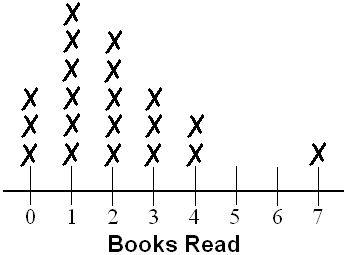Skill 4G
Line Plot

Mr. Mason gave his students a history test.
The scores are shown on this line plot.1. What is the range of these scores?

2. How many students received a test score higher than 96?3. Use the line plot above.  Which value is considered to be an outlier?